# Sum of the seventeen numbers

The sum of the 17 different natural numbers is 154. Determine the sum of the two largest ones.

s =  34

### Step-by-step explanation: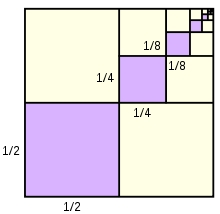Did you find an error or inaccuracy? Feel free to write us. Thank you!## Related math problems and questions:

• Find the sumFind the sum of all natural numbers from 1 and 100, which are divisible by 2 or 5
• Statistics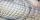The sum of all deviations from the arithmetic mean of the numerical sequence 4, 6, 51, 77, 90, 93, 95, 109, 113, 117 is:
• Consecutive numbersSum of ten consecutive numbers is 105. Determine these numbers (write first and last).
• Geometric progressionIn geometric progression, a1 = 7, q = 5. Find the condition for n to sum first n members is sn≤217.
• Sum of four numbers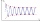The sum of four consecutive natural numbers is 114. Find them.
• Finite arithmetic sequenceHow many numbers should be inserted between the numbers 1 and 25 so that all numbers create a finite arithmetic sequence and that the sum of all members of this group is 117?
• NumbersDetermine the number of all positive integers less than 4183444 if each is divisible by 29, 7, 17. What is its sum?
• The sum 2The sum of five consecutive even integers is 150. Find the largest of the five integers. A.28 B.30 C.34 D.54 Show your solution and explain your answer.
• Series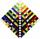Your task is express the sum of the following arithmetic series for n = 14: S(n) = 11 + 13 + 15 + 17 + ... + 2n+9 + 2n+11
• Two geometric progressions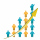Insert several numbers between numbers 6 and 384 so that they form with the given GP numbers and that the following applies: a) the sum of all numbers is 510 And for another GP to apply: b) the sum of entered numbers is -132 (These are two different geome
• Octahedron - sumOn each wall of a regular octahedron is written one of the numbers 1, 2, 3, 4, 5, 6, 7 and 8, wherein on different sides are different numbers. For each wall John make the sum of the numbers written of three adjacent walls. Thus got eight sums, which also
• DigitsShow that if x, y, z are 3 consecutive nonzero digits, zyx-xyz = 198, where zyx and xyz are three-digit numbers created from x, y, z.
• The sumThe sum of the squares of two immediately following natural numbers is 1201. Find these numbers.
• Diofant equation250x + 120y = 5640
• RemaindersIt is given a set of numbers { 170; 244; 299; 333; 351; 391; 423; 644 }. Divide this numbers by number 66 and determine set of remainders. As result write sum of this remainders.
• Cuboid wallsCalculate the cuboid volume if its different walls have an area of 195cm², 135cm², and 117cm².
• Gauss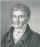Help little C.F. Gauss sum all the integers from 1 to 400.